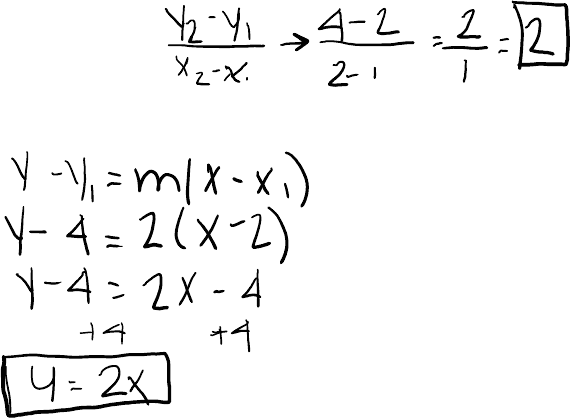# MATH221 Study Guide - Quiz Guide: Difference Quotient, Power Rule

158 views3 pagesQuiz is on sections 1.1-1.4
The slope of a straight line is y=mx + b
Where m is the slope of the line
Think of it as rYse/run (as in Y/x)
Get 2 points from the line
1.
2.
3.
Simply
4.
If given the graph of a straight line
Slope is defined as the change is Y over the change in X or rise/run
Y-Y1=m(x-x1)
Use this equation to determine the equation of the line (therefor
you need to know at least 2 points on the graph and the slope)
Find M (slope)
1.
If you have the data points (1,2) and (2, 4), you have everything
you need to solve the equation of the line:
Point-Slope form:
Section 1.1: The slope of a Straight Line
Substitute 1 data point and the slope in the equation:
2.
This is the SLOPE
Use the slope equation
Rate of Change:
The tangent line touches the curve at the specific point and extends as a
straight line with a constant slope
To find the slope of a curve we must draw a TANGENT LINE to the point.
Section 1.2: The Slope of a Curve at a Point:
Quiz 1 Study Guide:
Tuesday, September 11, 2018
9:55 PM
Quiz 1 Study Guide Page 1
Unlock document

This preview shows page 1 of the document.
Unlock all 3 pages and 3 million more documents.

## Document Summary

Section 1. 1: the slope of a straight line. The slope of a straight line is y=mx + b. Where m is the slope of the line. Slope is defined as the change is y over the change in x or rise/run. Think of it as ryse/run (as in y/x) If given the graph of a straight line. Use this equation to determine the equation of the line (therefor you need to know at least 2 points on the graph and the slope) If you have the data points (1,2) and (2, 4), you have everything you need to solve the equation of the line: Substitute 1 data point and the slope in the equation: Section 1. 2: the slope of a curve at a point: To find the slope of a curve we must draw a tangent line to the point. The tangent line touches the curve at the specific point and extends as a straight line with a constant slope.

## Get access